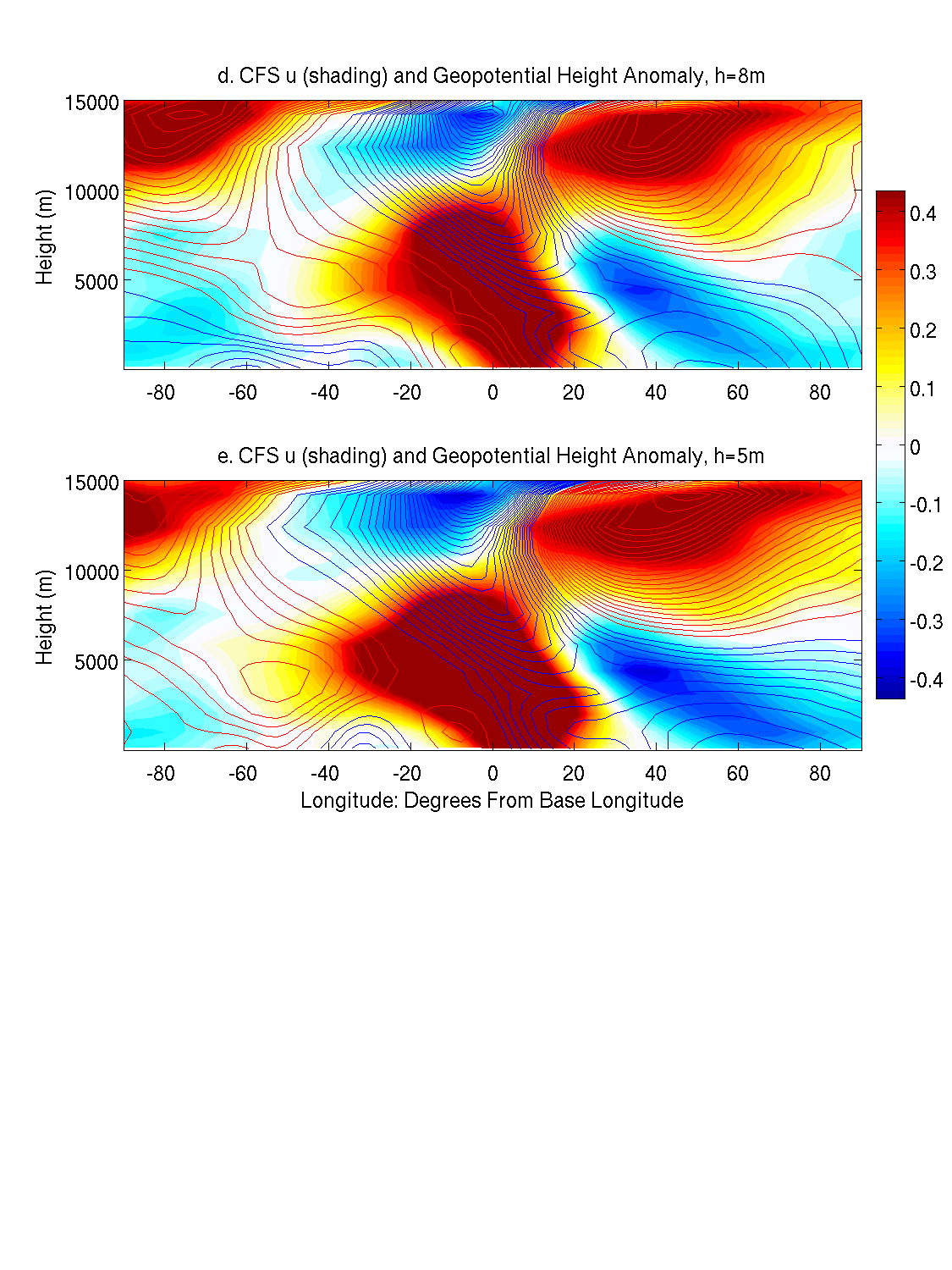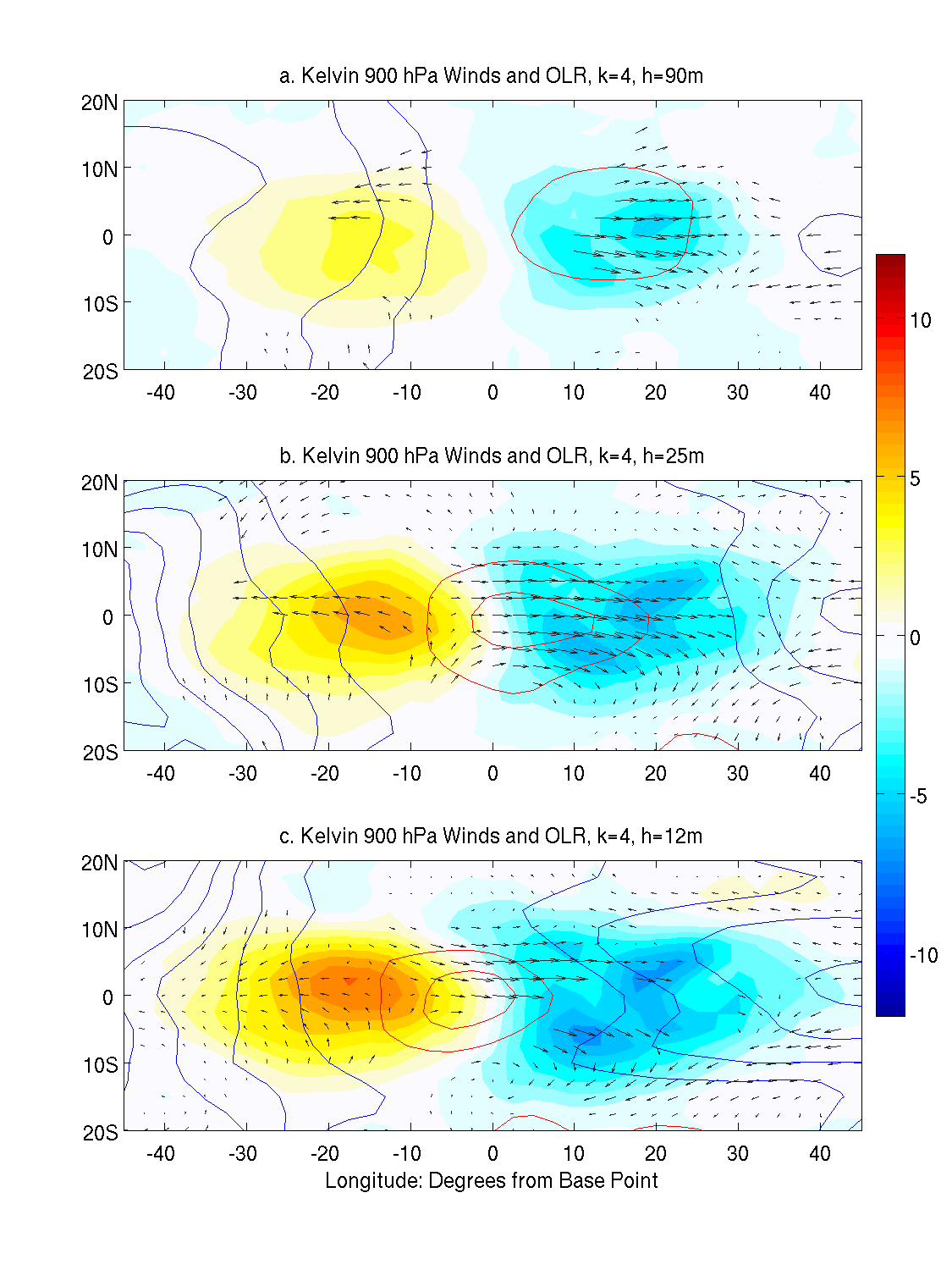# Observed Structure of Convectively Coupled Waves as a Function of Equivalent Depth: Kelvin Waves and the Madden Julian OscillationFigure 2. Longitude-height cross sections of regressed zonal wind anomalies (shading, ms-1) and geopotential height anomalies (contours, negative in blue, with an interval of 0.25m) for signals along the Kelvin wave dispersion curves at zonal wave number 4. Panels a-e represent results for equivalent depths of 90, 25, 12, 8, and 5m, respectively. The vertical axis is labeled in terms of regressed total geopotential height to facilitate measurement of vertical tilts. Positive longitude is represented as degrees east of the base points.Figure 3. Horizontal maps of regressed OLR anomalies (shading, Wm-2), geopotential height anomalies (positive in red, contour interval 0.15m), and wind anomalies at 900 hPa for signals along the Kelvin wave dispersion solutions for zonal wave number 4. Panels correspond to equivalent depths of 90, 25, 12, 8, and 5m, corresponding to the same panels of Fig. 2.Download 1.36 Mb.Share with your friends: